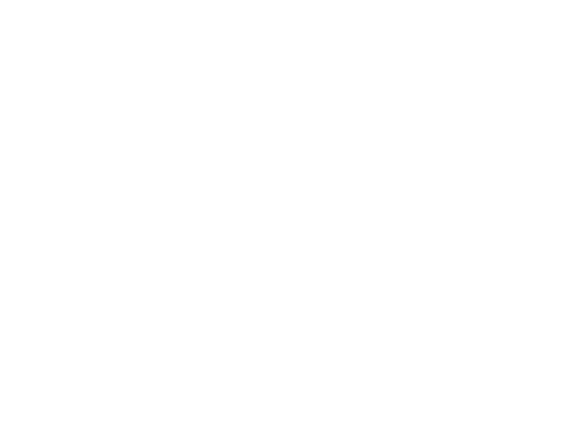Cody

Solution 1002513

Submitted on 8 Oct 2016 by uu tsi
This solution is locked. To view this solution, you need to provide a solution of the same size or smaller.

### Test Suite

Test Status Code Input and Output
1   Pass
for i = 1:100 a = counterfun; validatestring(char(a), {char(i)}) assert(isequal(a,i)) end

ans =  ans =  ans =  ans =  ans =  ans =  ans =  ans = ans = ans = ans = ans = ans = ans =  ans =  ans =  ans =  ans =  ans =  ans =  ans =  ans =  ans =  ans =  ans =  ans =  ans =  ans =  ans =  ans =  ans =  ans = ans = ! ans = " ans = # ans = \$ ans = % ans = & ans = ' ans = ( ans = ) ans = * ans = + ans = , ans = - ans = . ans = / ans = 0 ans = 1 ans = 2 ans = 3 ans = 4 ans = 5 ans = 6 ans = 7 ans = 8 ans = 9 ans = : ans = ; ans = < ans = = ans = > ans = ? ans = @ ans = A ans = B ans = C ans = D ans = E ans = F ans = G ans = H ans = I ans = J ans = K ans = L ans = M ans = N ans = O ans = P ans = Q ans = R ans = S ans = T ans = U ans = V ans = W ans = X ans = Y ans = Z ans = [ ans = \ ans = ] ans = ^ ans = _ ans = ` ans = a ans = b ans = c ans = d

``
``
``
``
``
``
``
``
``
``

### Community Treasure Hunt

Find the treasures in MATLAB Central and discover how the community can help you!

Start Hunting!